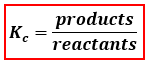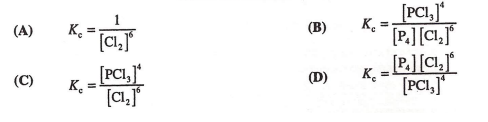Chemistry Practice Problems Equilibrium Expressions Practice Problems Solution: Consider this reaction.P4 (s) + 6 Cl2 (g) ⇌ 4 PCl3...

🤓 Based on our data, we think this question is relevant for Professor Bindell's class at UCF.

# Solution: Consider this reaction.P4 (s) + 6 Cl2 (g) ⇌ 4 PCl3 (g)What is the correct Kc expression for this reaction?

###### Problem

Consider this reaction.

P(s) + 6 Cl(g) ⇌ 4 PCl(g)

What is the correct Kc expression for this reaction?

###### Solution

Kc is the equilibrium expression of the concentrations in the reaction. The general form of an equilibrium expression is:Given reaction:

P4(s)  +  6 Cl2(g)  ⇌  4 PCl3(g)
(reactants)                  (products)

When writing equilibrium expressions:View Complete Written SolutionEquilibrium Expressions

Equilibrium Expressions

#### Q. Consider this reaction.2 C(s) + O2 (g) ⇌ 2 CO (g)What is the equilibrium expression for this reaction?

Solved • Tue May 10 2016 10:12:26 GMT-0400 (EDT)

Equilibrium Expressions

#### Q. Consider this reaction.2 SO3 (g) ⇌ 2 SO2 (g) + O2 (g)What is the correct Kp expression for this reaction?

Solved • Tue May 10 2016 09:59:46 GMT-0400 (EDT)

Equilibrium Expressions

#### Q. What is the relationship between the equilibrium constant (K c) of a reaction and the rate constants for the forward (kf) and reverse (k r) reactions?...

Solved • Mon May 09 2016 16:55:07 GMT-0400 (EDT)

Equilibrium Expressions

#### Q. The graph shows the varation of concentration with time for this reaction at 25°C3A(aq) ⇌ B(aq) + 2C(aq)What is the value of the equilibrium constant ...

Solved • Tue Apr 26 2016 14:24:44 GMT-0400 (EDT)